• 图像放大算法总结及MATLAB源程序1，插值算法(3种)：(1)最邻近插值(近邻取样法)：　最近插值的的思想很简单就是把这个非整数坐标作一个四舍五入，取最近的整数点坐标处的点的颜色。可见，最邻近插值简单且直观，但...
图像放大算法总结及MATLAB源程序1，插值算法(3种)：(1)最邻近插值(近邻取样法)：　　最近插值的的思想很简单就是把这个非整数坐标作一个四舍五入，取最近的整数点坐标处的点的颜色。可见，最邻近插值简单且直观，但得到的图像质量不高最邻近插值 = imread('F:\lena.jpg');%读取图像信息imshow(A); %显示原图title('原图128*128');Row = size(A,1); Col = size(A,2);%图像行数和列数nn=8;%放大倍数m = round(nn*Row);%求出变换后的坐标的最大值n = round(nn*Col);B = zeros(m,n,3);%定义变换后的图像for i = 1 : mfor j = 1 : nx = round(i/nn); y = round(j/nn);%最小临近法对图像进行插值if x==0  x = 1; endif y==0  y = 1; endif x>Row x = Row; endif y>Col y = Col;endB(i,j,:) = A(x,y,:);endendB = uint8(B);%将矩阵转换成8位无符号整数figure;imshow(B);title('最邻近插值法放大8倍1024*1024');运行程序后，原图如图1所示：图1用最邻近插值双线性内插：双线性内插对于一个目的像素，设置坐标通过反向变换得到的浮点坐标为(i+u,j+v)，其中i、j均为非负整数，u、v为[0,1)区间的浮点数，则这个像素得值 f(i+u,j+v) 可由原图像中坐标为 (i,j)、(i+1,j)、(i,j+1)、(i+1,j+1)所对应的周围四个像素的值决定，即：f(i+u,j+v) = (1-u)(1-v)f(i,j) + (1-u)vf(i,j+1) + u(1-v)f(i+1,j) + uvf(i+1,j+1)其中f(i,j)表示源图像(i,j)处的的像素值，以此类推这就是双线性内插值法。双线性内插值法计算量大，但缩放后图像质量高，不会出现像素值不连续的的情况。由于双线性插值具有低通滤波器的性质，使高频分量受损，所以可能会使图像轮廓在一定程度上变得模糊imresize( )来实现双线性内插值算法。双线性内插值算法的MATLAB源代码为：A=imread('F:\lena.jpg');imshow(A);title('原图128*128');C=imresize(A,8,'bilinear'); %双线性插值figure;imshow(C);title('双线性内插值法放大8倍1024*1024');程序运行后，原图如图3所示：图3双线性内插值法放大8倍后的图如图4所示：图4(3)双三次法法能够克服以上两种算法的不足，计算精度高，但计算大，考虑一个浮点坐标(i+u,j+v)周围的16个邻点目的像素值f(i+u,j+v)可由如下插值公式得到：f(i+u,j+v) = [A] * [B] * [C][A]=[ S(u + 1)　S(u + 0)　S(u - 1)　S(u - 2) ][C]=[ S(v + 1)　S(+ 0)　S( - 1)　S( - 2) ]T;而[B]是周围16个邻点组成的4*4的矩阵；S(x)是对 Sin(x*π)/x 的逼近imresize( )来实现双三次插值算法。MATLAB源代码为：A=imread('F:\lena.jpg'); %读取原图像D=imresize(A,8,'bicubic'); %双三次插值放大8倍figure;imshow(D);title('三次卷积法放大8倍1024*1024');MATLAB自带双三次插值法运行结果如图5所示：图5也可以自己编写双三次插值算法MATLAB代码如下：clc,clear;ff=imread('F:\lena.jpg');           %读取图像到ffk=8;                       %设置放大倍数[m,n,color]=size(ff);f=zeros(m,n);      %将彩色图像ff转换为黑白图像ffor i=1:mfor j=1:nf(i,j)=ff(i,j);endenda=f(1,:);c=f(m,:);             %将待插值图像矩阵前后各扩展两行两列,共扩展四行四列b=[f(1,1),f(1,1),f(:,1)',f(m,1),f(m,1)];d=
展开全文• 经典图像/视频缩放算法原理及MATLAB\Simulink实现1 图像/视频缩放介绍2 图像缩放的经典算法2.1 最近邻插值法2.2 双线性插值法3 图像缩放算法MATLAB实现3.1 最近邻算法实现3.2 双线性插值算法实现4 视频无极缩放的...
经典图像/视频缩放算法原理及MATLAB\Simulink实现1 图像/视频缩放介绍2 图像缩放的经典算法2.1 最近邻插值法2.2 双线性插值法3 图像缩放算法的MATLAB实现3.1 最近邻算法实现3.2 双线性插值算法实现4 视频无极缩放的Simulink实现
1 图像/视频缩放介绍
图像缩放，顾名思义是对图片进行缩小和放大。由于矢量图缩放过程不失真，因此这里所说的图像缩放指的是像素图。
视频是图像的时间序列，因此视频缩放就是对每帧图像的缩放，背后本质上还是图像缩放。
图像/视频缩放的作用不言而喻。比如小图片看不清楚，直接放大又显得模糊；大图片加载太慢，占用时间和网络流量，能不能以缩略图形式展示给用户；视频监控时对感兴趣目标放大，以观察细节；4K品质的视频太少，能否直接用视频处理技术提高海量存在的低分辨率视频的品质等等。
2 图像缩放的经典算法
第（1）部分已经说明视频缩放的本质是图像缩放，因此结合工程经验，只总结图像缩放的经典算法。
图像缩放算法的研究自动画和电影产生之初就有人开始研究，时至今日诞生了很多优秀的算法。综合来看，可以将图像缩放技术分为传统插值算法、基于边缘的插值算法和基于区域的插值算法。本文只总结传统插值算法：最近邻插值法、双线性插值法。
2.1 最近邻插值法
图像缩放最容易想到的是按照比例缩放，则像素点也按照比例填充即可，找不到原像的像素点可以采用近邻算法来四舍五入。由此诞生了最简单、最早得到应用、也最广为人知的最近邻插值法（当图像缩小整数倍时，最近邻插值法会退变为按比例填充，我称之为比例填充法）。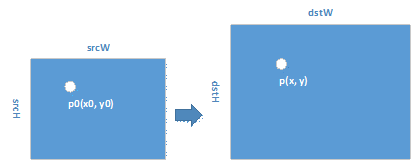原理很简单：可以按照函数或者映射的思想来看这个问题，将原图当做原像，将缩放后的图像当做像。那么原像中的像素点，根据比例算法映射到像中的像素点的过程就是图像缩放的过程。
假设原像source picture中的像素点位置为 sx、sy，像素为s_rgb；像target picture中的像素点位置为 tx、ty，像素为t_rgb。缩放比例为pic_ratio。
则
	sx = tx / pic_ratio;
sy = ty / pic_ratio;
if sx - floor(sx) > =0.5	 %% sy is similar to sx
sx = sx + 1;
else
sx = sx;		%  attentation to the bounding of pixel matrix
t_rgb = s_rgb;

2.2 双线性插值法
最邻近插值是当求得p0后，直接找其邻近的点p1, p2, p3, p4中的一个的像素值作为目标点的像素；而双线性插值，则是根据p0点与周围4点(p1, p2, p3, p4)距离关系计算目标点的像素值。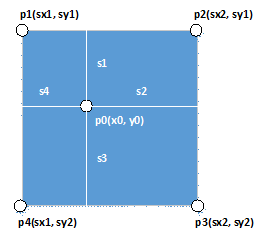通过计算得到的原始点为p0(x0, y0)，则其4周的点分别为：
x0的可能取值为：sx1 = (int)x0, sx2 = sx1 + 1
y0的可能取值为：sy1 = (int)y0, sy2 = sy1 + 1
图2中：
s1 = y0 – sy1
s2 = sx2 – x0
s3 = 1.0 – s1
s4 = 1.0 – s2
假设p1, p2, p3, p4的像素值分别为v1, v2, v3, v4，
距离周围像素点越近，近的像素点所占权重应该越大，则双线性插值计算p0点像素值v0公式为：
v0 = v1*(1-s1)(1-s4) + v2(1-s1)(1-s2) + v3(1-s2)(1-s3) + v4(1-s3)*(1-s4)
3 图像缩放算法的MATLAB实现
3.1 最近邻算法实现
代码
clear;
clc;

% target pic's resize ratio
target_ratio = 0.2;

% get the height,width,rgb of image
[h,w,c] = size(imagedata);

% [th,tw,c] is the target pic's size
target_size = [h,w] * target_ratio;
th = floor(target_size(1));
tw = floor(target_size(2));
tc = c;
target_pic = uint8(zeros(th,tw,tc));

% solve target pic's rgb,
% x0, y0 is the point in source pic; i, j is the point in target pic
for i = 1:th - 1
for j = 1:tw - 1
x0 = i / target_ratio;
y0 = j / target_ratio;
sx = 0;
sy = 0;
if x0 - floor(x0) >= 0.5
sx = floor(x0) + 1;
else
sx = floor(x0);
end
if y0 - floor(y0) >= 0.5
sy = floor(y0) + 1;
else
sy = floor(y0);
end

if sx == 0
sx = 1;
end
if sx > h
sx = h;
end
if sy == 0
sy = 1;
end
if sy > w
sy = w;
end
target_pic(i,j,1) = imagedata(sx,sy,1);
target_pic(i,j,2) = imagedata(sx,sy,2);
target_pic(i,j,3) = imagedata(sx,sy,3);
end
end

% save target pic
% target_pic = image(target_pic);
target_pic_name = 'target_pic_linjin.jpg';
if(exist(target_pic_name,'file'))
delete(target_pic_name);
end
imwrite(target_pic,'target_pic_linjin.jpg');

效果效果还是很明显的，最近邻在比例为0.2时缩放的效果不清晰，实际上所有缩放算法只有在比例较小或者较大时才能看出效果，比例接近1差别就很小了。
3.2 双线性插值算法实现
代码
clear;
clc;

% target pic's resize ratio
target_ratio = 0.2;

% get the height,width,rgb of image
[h,w,c] = size(imagedata);

% [th,tw,c] is the target pic's size
target_size = [h,w] * target_ratio;
th = floor(target_size(1));
tw = floor(target_size(2));
tc = c;
target_pic = uint8(zeros(th,tw,tc));

% solve target pic's rgb,
% x0, y0 is the point in source pic; i, j is the point in target pic
for i = 1:th - 1
for j = 1:tw - 1
x0 = i / target_ratio;
y0 = j / target_ratio;
sx1 = floor(x0);
if sx1 == 0
sx1 = 1;
end
sx2 = sx1 + 1;
if sx2 > h
sx2 = h;
end
sy1 = floor(y0);
if sy1 == 0
sy1 = 1;
end
sy2 = sy1 + 1;
if sy2 > w
sy2 = w;
end
s1 = abs(y0 - sy1);
s2 = abs(sx2 - x0);
s3 = abs(1.0 - s1);
s4 = abs(1.0 - s2);
target_pic(i,j,1) = floor(imagedata(sx1,sy1,1) * (1 - s1) * (1 - s4) + imagedata(sx2,sy1,1) * (1 - s1) * (1 - s2) + imagedata(sx2,sy2,1) * (1 - s2) * (1 - s3) + imagedata(sx1,sy2,1) * (1 - s3) * (1 - s4));
target_pic(i,j,2) = floor(imagedata(sx1,sy1,2) * (1 - s1) * (1 - s4) + imagedata(sx2,sy1,2) * (1 - s1) * (1 - s2) + imagedata(sx2,sy2,2) * (1 - s2) * (1 - s3) + imagedata(sx1,sy2,2) * (1 - s3) * (1 - s4));
target_pic(i,j,3) = floor(imagedata(sx1,sy1,3) * (1 - s1) * (1 - s4) + imagedata(sx2,sy1,3) * (1 - s1) * (1 - s2) + imagedata(sx2,sy2,3) * (1 - s2) * (1 - s3) + imagedata(sx1,sy2,3) * (1 - s3) * (1 - s4));
end
end

% save target pic
% target_pic = image(target_pic);
target_pic_name = 'target_pic.jpg';
if(exist(target_pic_name,'file'))
delete(target_pic_name);
end
imwrite(target_pic,'target_pic.jpg');

缩放效果也不是很好。可能选用的图片分辨率太高，两种算法在同一个缩放比例下效果不是很明显，应该用条形图作对比的。代码
function y = image_resize_fcn(raw_mage)
%#codegen
imagedata = raw_mage;

% target pic's resize ratio
target_ratio = 0.5;

% get the height,width,rgb of image
[h,w,c] = size(imagedata);

% [th,tw,c] is the target pic's size
target_size = [h,w] * target_ratio;
th = floor(target_size(1));
tw = floor(target_size(2));
tc = c;
target_pic = uint8(zeros(th,tw,tc));

% solve target pic's rgb,
% x0, y0 is the point in source pic; i, j is the point in target pic
for i = 1:th - 1
for j = 1:tw - 1
x0 = i / target_ratio;
y0 = j / target_ratio;
sx1 = floor(x0);
if sx1 == 0
sx1 = 1;
end
sx2 = sx1 + 1;
if sx2 > h
sx2 = h;
end
sy1 = floor(y0);
if sy1 == 0
sy1 = 1;
end
sy2 = sy1 + 1;
if sy2 > w
sy2 = w;
end
s1 = abs(y0 - sy1);
s2 = abs(sx2 - x0);
s3 = abs(1.0 - s1);
s4 = abs(1.0 - s2);
target_pic(i,j,1) = floor(imagedata(sx1,sy1,1) * (1 - s1) * (1 - s4) + imagedata(sx2,sy1,1) * (1 - s1) * (1 - s2) + imagedata(sx2,sy2,1) * (1 - s2) * (1 - s3) + imagedata(sx1,sy2,1) * (1 - s3) * (1 - s4));
target_pic(i,j,2) = floor(imagedata(sx1,sy1,2) * (1 - s1) * (1 - s4) + imagedata(sx2,sy1,2) * (1 - s1) * (1 - s2) + imagedata(sx2,sy2,2) * (1 - s2) * (1 - s3) + imagedata(sx1,sy2,2) * (1 - s3) * (1 - s4));
target_pic(i,j,3) = floor(imagedata(sx1,sy1,3) * (1 - s1) * (1 - s4) + imagedata(sx2,sy1,3) * (1 - s1) * (1 - s2) + imagedata(sx2,sy2,3) * (1 - s2) * (1 - s3) + imagedata(sx1,sy2,3) * (1 - s3) * (1 - s4));
end
end

% save target pic
y = target_pic;
end



展开全文• % matlab库函数，调用imresize可以重新生成不同尺寸的图片   cubic_factor函数 % 距离系数计算公式 % ┏ 1 - 2 *Abs(x)^ 2 +Abs(x)^ 3 　, 0 (x)< 1 % S(x)=｛ 4 - 8 *Abs(x)+ 5 *...
http://www.cnblogs.com/xy-kidult/p/3404035.html

主要有两个代码，一个是主代码，包括注释，另一个是cubic_factor函数，用于计算距离系数的。clear;
close all;
clc;

CONST=50;
bwimg=im2bw(A);
resize_img=[];
[srcWidth srcHeight]=size(bwimg);

% %
% 以下算法思路参考http://blog.csdn.net/ArtX/article/details/1540539和http://blog.csdn.net/qiqi5521/article/details/2207562这两篇博客，谢谢博主。
% % 最近邻
% % 思想是根据 srcWidth/dstWidth = srcX/dstY，最后再对srcX取整
% % 缺点是精度不够，严重失真
%
% dstWidth=srcWidth+CONST*2;
% dstHeight=srcHeight+CONST*2;
% resize_img=zeros(dstWidth,dstHeight);
%
% % 像素变化公式计算公式
% for i=1:dstWidth
%     for j=1:dstHeight
%     src_i=i*(srcWidth/dstWidth);
%     src_j=j*(srcHeight/dstHeight);
%     resize_img(i,j)=bwimg(round(src_i),round(src_j)); % round四舍五入
%     end
% end
%
% figure,imshow(bwimg);
% figure,imshow(resize_img)

% % 双线性内插值算法
% % 聪明的方法
% % 最近邻法中根据 srcWidth/dstWidth = srcX/dstY，可以倒退出一个srcX的坐标值，但是这个值是浮点数，
% % 这个浮点数提供了一些信息，它离它在原始图像四周的像素值的关系，根据这个关系，计算一个更接近的dstX，
% % 然后再填充目标图像
% % 公式：
% % f(i+u,j+v)=(1-u)(1-v)f(i,j)+(1-u)vf(i,j+1)+u(1-v)f(i+1,j)+uvf(i+1,j+1)
% % i,j 为浮点坐标的整数部分； u,v为浮点坐标的小数部分
% % 优点：缩放后图像质量高
% % 缺点： 计算量大，双线性插值具有低通滤波器的性质，使高频分量受损，图像轮廓在一定程度上变模糊
%
% dstWidth=srcWidth+CONST*2;
% dstHeight=srcHeight+CONST*2;
% resize_img=zeros(dstWidth,dstHeight);
%
% % 像素变化公式计算公式
% for i=1:dstWidth-1
%     for j=1:dstHeight-1
%     src_i=i*(srcWidth/dstWidth);
%     src_j=j*(srcHeight/dstHeight);
%     src_ii=fix(src_i);
%     src_iu=src_i - src_ii; % none fix part
%     src_jj=fix(src_j);
%     src_jv=src_j - src_jj;
%     if src_ii == 0
%         src_ii=src_ii+1;
%     end
%     if src_jj ==0
%         src_jj=src_jj+1;
%     end
%     resize_img(i,j)=(1-src_iu)*(1-src_jv)*bwimg(src_ii,src_jj)+(1-src_iu)*src_jv*bwimg(src_ii,src_jj+1)+src_iu*(1-src_jv)*bwimg(src_ii+1,src_jj)...
%         +src_iu*src_jv*bwimg(src_ii+1,src_jj+1);
%     end
% end
% figure,imshow(bwimg);
% figure,imshow(resize_img)

% 三次卷积法
% 三次卷积法计算精度高，但计算亮大，思路是根据一个浮点坐标(i+u,j+v)周围的16个邻点，由下列公式得出目的像素值f(i+u,j+v)：
%
% 　　　　f(i+u,j+v) = [A] * [B] * [C]
%
% [A]=[ S(u + 1)　S(u + 0)　S(u - 1)　S(u - 2) ]
%
% 　　┏ f(i-1, j-1)　f(i-1, j+0)　f(i-1, j+1)　f(i-1, j+2) ┓
% [B]=┃ f(i+0, j-1)　f(i+0, j+0)　f(i+0, j+1)　f(i+0, j+2) ┃
% 　　┃ f(i+1, j-1)　f(i+1, j+0)　f(i+1, j+1)　f(i+1, j+2) ┃
% 　　┗ f(i+2, j-1)　f(i+2, j+0)　f(i+2, j+1)　f(i+2, j+2) ┛
%
% 　　┏ S(v + 1) ┓
% [C]=┃ S(v + 0) ┃
% 　　┃ S(v - 1) ┃
% 　　┗ S(v - 2) ┛
%
% 　　 ┏ 1-2*Abs(x)^2+Abs(x)^3　　　　　 , 0<=Abs(x)<1
% S(x)=｛ 4-8*Abs(x)+5*Abs(x)^2-Abs(x)^3　, 1<=Abs(x)<2
% 　　 ┗ 0　　　　　　　　　　　　　　　 , Abs(x)>=2
% 有人说，S(x)是对 Sin(x*Pi)/x
% 的逼近（Pi是圆周率——π），通过极限计算，可以知道Sin(x*Pi)/x的范围在0~pi之间，无法理解为什么要这样设置S(x）
% 但上述求A、B、C的公式还是可以理解，根据双线性插值的思想，也就是根据浮点数在原始坐标中与邻近像素的距离远近来决定原始坐标在目的坐标中所占的比重

dstWidth=srcWidth-CONST*2;
dstHeight=srcHeight-CONST*2;
resize_img=zeros(dstWidth,dstHeight);
A=[];B=[];C=[];

% 像素变化公式计算公式
for i=3:dstWidth-2
for j=3:dstHeight-2
src_i=i*(srcWidth/dstWidth);
src_j=j*(srcHeight/dstHeight);
src_ii=fix(src_i); % 整数部分
src_iu=src_i - src_ii; % 小数部分
src_jj=fix(src_j);
src_jv=src_j - src_jj;

A=[ cubic_factor(src_iu+1) cubic_factor(src_iu) cubic_factor(src_iu-1) cubic_factor(src_iu-2) ];
C=[ cubic_factor(src_jv+1); cubic_factor(src_jv); cubic_factor(src_jv-1); cubic_factor(src_jv-2) ];
B=[ bwimg(src_ii -1,src_jj -1) bwimg(src_ii-1,src_jj) bwimg(src_ii-1,src_jj+1) bwimg(src_ii-1,src_jj+2);...
bwimg(src_ii,src_jj-1) bwimg(src_ii,src_jj) bwimg(src_ii,src_jj+1) bwimg(src_ii,src_jj+2); ...
bwimg(src_ii+1,src_jj-1) bwimg(src_ii+1,src_jj) bwimg(src_ii+1,src_jj+1) bwimg(src_ii+1,src_jj+2); ...
bwimg(src_ii+2,src_jj-1) bwimg(src_ii+2,src_jj) bwimg(src_ii+2,src_jj+1) bwimg(src_ii+2,src_jj+2) ];

resize_img(i,j)=A*B*C;

end
end

figure,imshow(bwimg);
figure,imshow(resize_img)

% dstimg=imresize(srcimg,4,'bicubic'); % matlab库函数，调用imresize可以重新生成不同尺寸的图片cubic_factor函数% 距离系数计算公式
% 　　 ┏ 1-2*Abs(x)^2+Abs(x)^3　　　　　 , 0<=Abs(x)<1
% S(x)=｛ 4-8*Abs(x)+5*Abs(x)^2-Abs(x)^3　, 1<=Abs(x)<2
% 　　 ┗ 0　　　　　　　　　　　　　　　 , Abs(x)>=2
function S=cubic_factor(x)

if 0<=abs(x)<1
S=1-2.*abs(x).^2+abs(x).^3;
elseif 1<=abs(x)<2
S=4-8.*abs(x)+5.*abs(x).^2-abs(x).^3;
else
S=0;
end上面代码有一个问题，”最右边和最下边的像素实际上并没有参与计算“，似乎是由于最初的中心点选择方法引起的，网上有一个人提出了解决方案(http://handspeaker.iteye.com/blog/1545126)，看了一下，大概了解他的想法，试验之后，没有效果，可能我理解错了，下次再来解决这个问题并修改代码吧。

展开全文• Write a function Reduce / Expand that has an image as an input and the output should be re-sampled copy of half / twice the width and height of the input image.
• 实现图像缩放功能的Matlab插值算法研究与比较.pdf
• 本文选择了一种新颖的图像缩放算法进行FPGA硬件实现。该算法基于奇偶分解的思想，具有复杂度低、硬件需求小和缩放效果良好等突出优点。首先利用MATLAB对该算法进行了功能验证，然后用缩放耗时、PSNR、边缘模糊等级和...
• ## 图像缩放算法小结

万次阅读 多人点赞 2019-04-10 09:33:18
双线性插值法效果要好于最近邻插值，只是计算量稍大一些，算法复杂些，程序运行时间也稍长些，但缩放图像质量高，基本克服了最近邻插值灰度值不连续的特点，因为它考虑了待测采样点周围四个直接邻点对该采样点的...
参考网址：
https://blog.csdn.net/lfz_sau/article/details/51366700
http://www.cnblogs.com/blfshiye/p/4823027.html
https://en.wikipedia.org/wiki/Lanczos_resampling
https://wenku.baidu.com/view/22b38b70a417866fb84a8ea1.html
https://en.wikipedia.org/wiki/Pixel-art_scaling_algorithms
https://blog.csdn.net/u011630458/article/details/69524582
图像缩放的基本原理就是根据原图像的像素值通过一定的规则计算得到目标图像的像素值。
图像缩放的两个关键问题是应该选取原图像中哪些像素值，以及如何确定选取的像素值的权重。
图像缩放的常见问题是产生锯齿（缩小）或者模糊（放大）。
缩放图像是二维的尺度伸缩变换，目标图像的像素值从原图像得到。
令h_step = H/h,		w_step = W/w，目标图中相邻像素的距离就转换为了原图中的h_step和w_step（通常不是整数）。
此外，原图和目标图的第一个像素重合。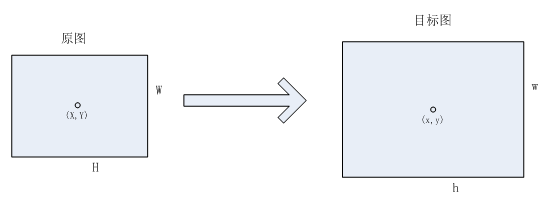最近邻算法
在待求像素的（待插值图的）四邻像素中，将距离待求像素最近的邻像素灰度赋给待求像素。
见下图，设u为待求像素与四邻像素的左上点的水平坐标差，v为待求像素与四邻像素的左上点的垂直坐标差。将待求像素在待插值图中的坐标位置进行四舍五入处理，对应坐标的像素值即为待求像素的值。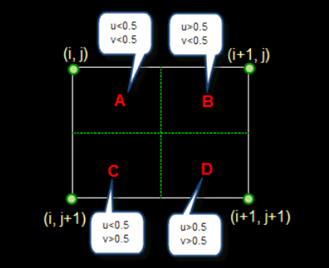优点：
最常见，最通用的算法之一，计算量很小，算法简单，因此运算速度较快。
缺点：
效果不好，放大图像时会出现严重的马赛克，缩小图像则会严重失真。它仅使用离待测采样点最近的像素的灰度值作为该采样点的灰度值，而没考虑其他相邻像素点的影响，因而重新采样后灰度值有明显的不连续性，图像质量损失较大，会产生明显的马赛克和锯齿现象。
Bilinear算法
双线性插值即在两个方向分别进行一次线性插值，通过四个相邻像素插值得到待求像素。
见下图，已知Q11，Q12，Q21，Q22为原图中的四邻像素，P点为待求像素。
图中所示的双线性插值步骤：
（1）通过Q12，Q22线性插值得到R2，通过Q11，Q21线性插值得到R1；
（2）通过R1，R2线性插值得到P.
图中所示的双线性插值先进行了水平方向的插值，再进行垂直方向上的插值，也可以先垂直插值再水平插值。插值方向的顺序不影响最终的结果。
双线性插值的公式为：
f(x,y)=f(0,0)(1-x)(1-y)+f(1,0)x(1-y)+f(0,1)(1-x)y+f(1,1)xy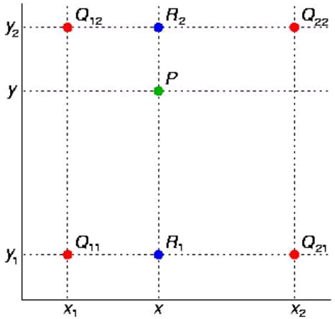优点：
最常见，最通用的算法之一，效果比最近邻插值法好。计算量较小，运算速度较快。图像连续性较好。双线性插值法效果要好于最近邻插值，只是计算量稍大一些，算法复杂些，程序运行时间也稍长些，但缩放后图像质量高，基本克服了最近邻插值灰度值不连续的特点，因为它考虑了待测采样点周围四个直接邻点对该采样点的相关性影响。
缺点：
放大时图像较为模糊，细节损失较严重。它仅考虑待测样点周围四个直接邻点灰度值的影响, 而未考虑到各邻点间灰度值变化率的影响, 因此具有低通滤波器的性质, 从而导致缩放后图像的高频分量受到损失, 图像边缘在一定程度上变得较为模糊。用此方法缩放后的输出图像与输入图像相比, 存在由于插值函数设计考虑不周而产生的图像质量受损与计算精度不高的问题。
Bicubic算法
在数值分析这个数学分支中，双三次插值（英语：Bicubic interpolation）是二维空间中最常用的插值方法。在这种方法中，函数f在点 (x,y) 的值可以通过矩形网格中最近的十六个采样点的加权平均得到，在这里需要使用两个多项式插值三次函数，每个方向使用一个。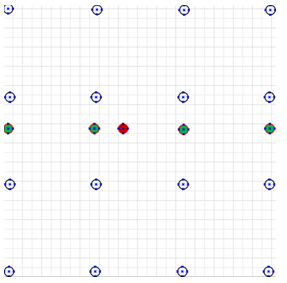通过双三次插值可以得到一个连续的插值函数，它的一阶偏导数连续，并且交叉导数处处连续。
双三次插值的计算公式：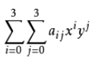双三次插值过程类似于双线性插值，分水平插值和垂直插值两个步骤，但双三次插值涉及的像素数更多(4x4)。
计算系数 的过程依赖于插值数据的特性。如果已知插值函数的导数，常用的方法就是使用四个顶点的高度以及每个顶点的三个导数。一阶导数h’x与h’y表示 x 与 y 方向的表面斜率，二阶相互导数h’’xy表示同时在 x 与 y 方向的斜率。这些值可以通过分别连续对 x 与 y 向量取微分得到。对于网格单元的每个顶点，将局部坐标（0,0, 1,0, 0,1 和 1,1) 带入这些方程，再解这 16 个方程。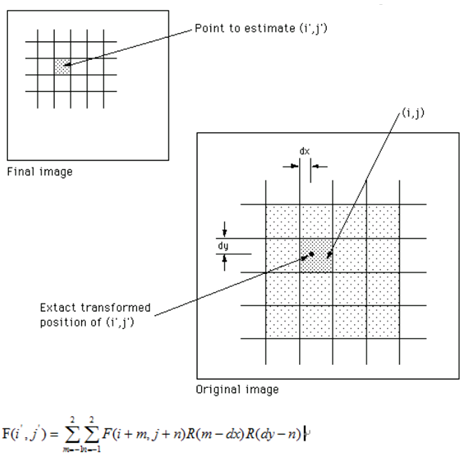上图公式中的R(x)表示插值采样函数，可以根据需要选择不同的采样函数。常见的有基于三角采样函数、Bell分布采样函数和B样条采样函数。下面介绍这三种常见的双三次插值采样函数。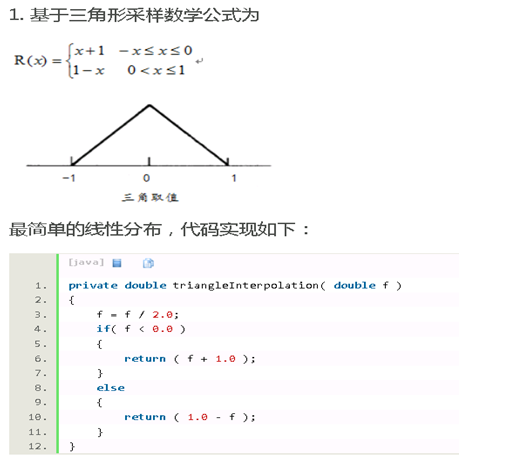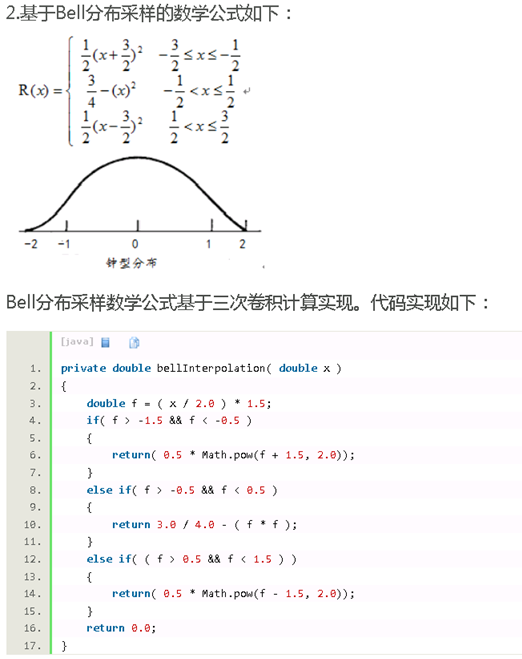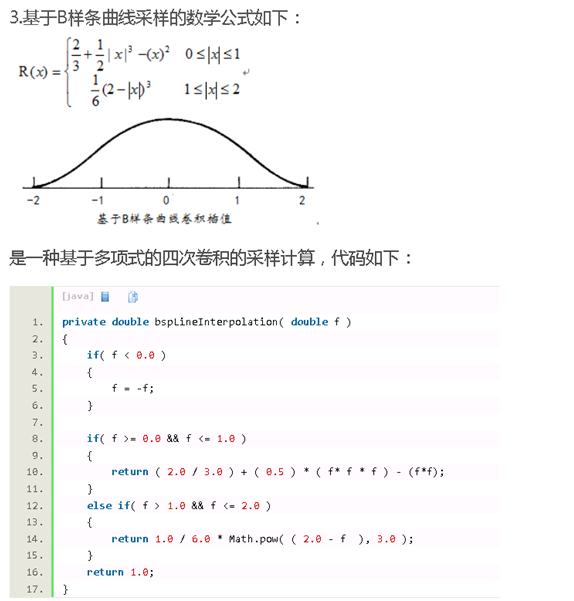优点：
立方卷积插值计算量最大，算法也是最为复杂的。在几何运算中，双线性内插法的平滑作用可能会使图像的细节产生退化，在进行放大处理时，这种影响更为明显。在其他应用中，双线性插值的斜率不连续性会产生不希望的结果。立方卷积插值不仅考虑到周围四个直接相邻像素点灰度值的影响，还考虑到它们灰度值变化率的影响。因此克服了最近邻算法和双线性算法的不足之处，能够产生比双线性插值更为平滑的边缘，计算精度很高，处理后的图像像质损失最少，效果是最佳的。
缺点：
立方卷积插值计算量较大，算法相较双线性算法更为复杂。
Lanczos算法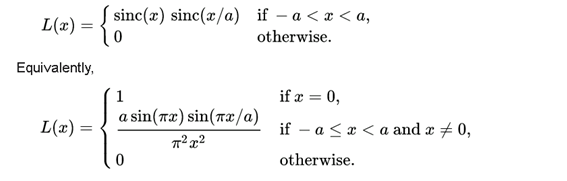a的典型值是2或3，得到的图像在抗锯齿和保留锐利边缘的效果最佳。下图是a=2和a=3时对应的函数图像。a = 2适合缩小图像，a = 3适合放大图像。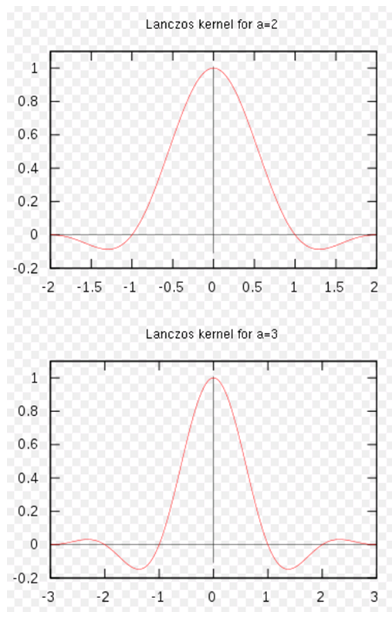类似于双三次插值，对4x4的像素卷积处理，得到待求像素。
优点：
得到的图像在抗锯齿和保留锐利边缘的效果较好。
缺点：
相当于立方卷积插值，计算量较大，算法相较双线性算法更为复杂。
NEDI算法
New Edge-Directed Interpolation，利用局部协方差特性来推导最佳线性MMSE预测的预测系数。由于低分辨率和高分辨率图像在边缘处具有相近的边缘信息，从而可以用低分辨率的协方差特性来得到高分辨率的预测系数。
插值方法：通过4邻域点的值乘上各自的权重值来得到插值点的值（45°或135°方向上的点可看作旋转后的4邻域）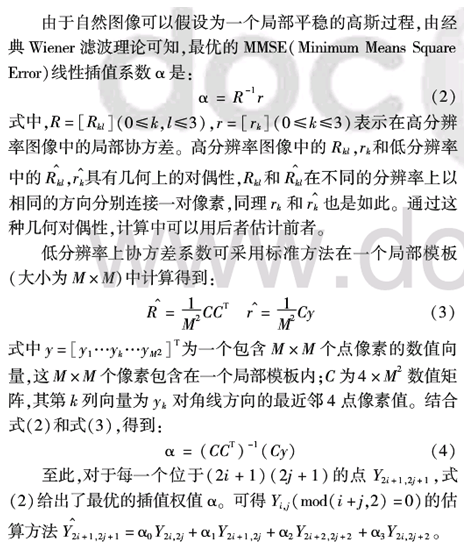（摘自论文，出处暂时没找到。）
插值过程说明如下：
（1）待插值点为O’点（偶行偶列）。图中□ABCD与□A’B’C’D’结构相似，假设它们具有相同的边缘信息。由于O点与A,B,C,D具有某种相关性，则对应地O’点与A’，B’，C’，D’有同样的相关性。因而可以通过求O点和A, B, C, D的协方差来得到A’, B’, C’, D’的权重值，进而插值得到O’的值。
分别以A, B, C, D, O为中心取7x7的矩阵，计算A, B, C, D 与 O 的相关性，得到系数a, 公式为a=inv(C’ *C) *(C’ *r)
其中矩阵C是由以点A, B, C, D为中心的7x7矩阵的像素值组成的， r表示点由以点O为中心的7x7矩阵的像素值组成的。得到的结果a表示A’, B’, C’, D’的权重值。
注意: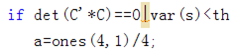这两个条件说明A, B, C, D与O线性无关或处于平坦区域，因而令A’, B’, C’, D’的权重值均为1/4。此外，若计算结果超出0~255的范围，说明计算结果异常，为保证不出现坏点，也令A’, B’, C’, D’的权重值均为1/4.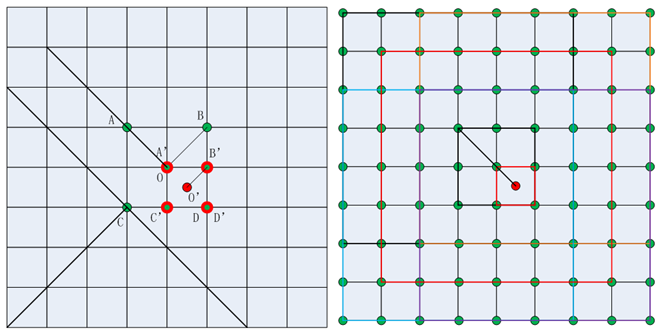（2）待插值点为图中的浅绿色点或紫色点（偶行奇列，奇行偶列）。原理同前，矩阵取点方向为逆时针45°。如下图所示，取大小为6x6的框。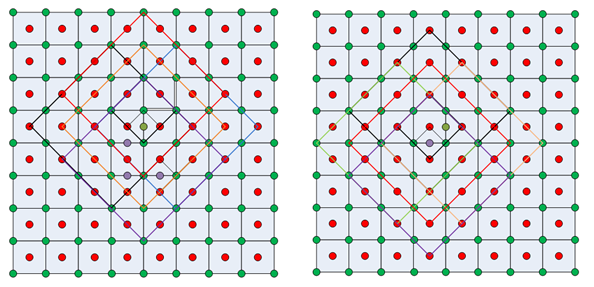优点：
根据统计信息进行插值，细节保持较好。
缺点：
算法复杂，计算量大，运算速度较慢。
EP2X算法
EPX(Eric’s Pixel Expansion)是1992年Eric Johnston为了将SCUMM游戏放大到IBM PC上而设计的。算法具体实现如下所示：
左下图为放大前的像素图，P为放大前的取样像素，ABCD为放大2倍后，取代P的四个子像素。
A = P; B = P; C = P; D = P;
若2, 4的值相同(R/G/B)，则A = 2;
若2, 6的值相同(R/G/B)，则B = 6;
若4, 8的值相同(R/G/B)，则C = 4;
若6, 8的值相同(R/G/B)，则D = 8;
若2, 4, 6, 8有三个以上相同，则A = B = C = D = P.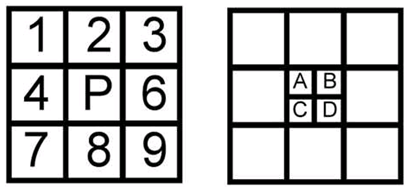基于EPX的改进算法Eagle：
A = P; B = P; C = P; D = P;
若1, 2, 4的值相同(R/G/B), 则A = 1;
若2, 3, 6的值相同(R/G/B), 则B = 3;
若4, 7, 8的值相同(R/G/B), 则C = 7;
若6, 8, 9的值相同(R/G/B), 则D = 9.
上述EPX 2x和Eagle 2x算法在RGB域已用MATLAB实现，若要对YUV域应用这两种算法，建议仅对Y分量实现。
优点：
算法简单，计算量较小，运算速度较快。边缘保持较好。
缺点：
适用于内容简单的图像，例如UI图，对于情景较为复杂的图像容易产生坏点。
HQX算法
HQX系列算法原理：
通过比较输入图像中的像素点与其8邻域像素点的值，标记邻域点为近或者远(0或1)，并使用一个预先生成的查找表格确定输入像素点相应输出的4、9或16个点的合适的权重。其中hq3x算法对斜线具有较好的抗锯齿作用。
下面具体介绍HQ2X算法。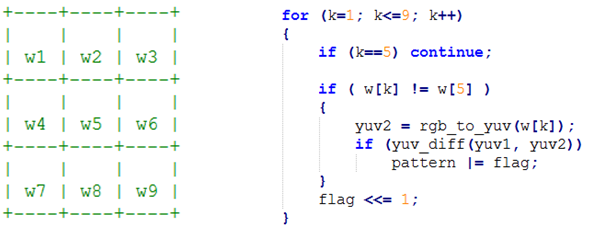在原图中取如图所示3x3的像素，计算w5和其8邻像素的差的绝对值，大于48则为1，否则为0.
flag = f(w1)<<7 +f(w2)<<6 + f(w3)<<5 + f(w4)<<4 + f(w6)<<3 + f(w7)<< 2 + f(w8)<<1 + f(w9)<<0
易知，flag的取值为0~255.
HQ2X算法里原图中的1个像素映射到目标图中的4个像素——PIXEL00，PIXEL01，PIXEL10，PIXEL11。
插值计算函数有：
Interp1(c1, c2) : ret = (c13 + c2)>>2;
Interp2(c1,c2,c3) : ret = (c12 + c2 + c3)>>2;
Interp3(c1,c2) : ret = (c17 + c2)>>3;
Interp4(c1,c2,c3): ret = (c12 + c27 + c37)>>4;
Interp5(c1,c2) : ret = (c1 + c2)>>1;
Interp6(c1,c2,c3) : ret = (c15 + c22 + c3)>>3;
Interp7(c1,c2,c3) : ret = (c16 + c2 + c3)>>3;
Interp8(c1,c2) : ret = (c15 + c23)>>3;
Interp9(c1,c2,c3) : ret = (c12 + c23 + c33)>>3;
Interp10(c1,c2,c3) : ret = (c1*14 + c2 + c3)>>4.
HQ2X用到了其中的Interp1, Interp2, Interp6, Interp7, Interp9, Interp10.具体算法情况参见C代码，共有161种情况。
优点：
边界清晰，对比度高，细节保持较好。
缺点：
斜边或者有弧度的地方的连续性不好，有明显的锯齿。
先由LR(low resolution)图像经简单的upscaling得到HR-tmp(high resolution)图像，再用训练得到的滤波模板处理，得到最终的HR图像。
RAISR的MATLAB代码组成部分：training和testing。
Training部分：
（1）已知original HR图，将其长宽均缩小到1/k，再通过cheap upscaling（如bilinear, bicubic等）放大到原尺寸，得到tmp HR图；
（2）在tmp HR图中取固定大小的patch，利用最小二乘法求解出滤波模板H。则可通过tmp HR图估计original HR图中的像素；
， 公式中的Ai指的是tmp HR图像中的patch，h是滤波模板，bi是original HR中相应的像素。直接求此公式的计算复杂度较高，所以转换成如下公式（这里从二维降为一维计算，简化了计算复杂度）；
， 其中 ，即将上述公式左乘A的转置。
（3）考虑到patch不同旋转角度的线性滤波模板有所不同，且它们可以统一到一个模板中，故（2）中的A取了patch旋转90°，垂直翻转和中心对称三种情况进行累加计算（MATLAB代码仅有三种，论文中说了四种方向）；
（4）计算patch的idx, 根据梯度angle, strength, coherence（与水平和垂直的梯度有关，具体计算参见代码或论文）计算得到（详见代码）；
（5）计算当前像素的type，与缩放倍数k有关，type的取值范围是1~k^2，行和列号可被k整除的type为1，在k*k的块内从左到右、从上到下type递增；
（6）将（3）中计算得到的滤波模板h保存到对应的idx, type类中；
（7）对大量图像重复上述步骤。
Testing部分：
（1）已知original HR图，将其长宽均缩小到1/k，再通过cheap upscaling（如bilinear, bicubic等）放大到原尺寸，得到tmp HR图；
（2）在tmp HR图中取固定大小的patch；
（3）计算patch的idx, 根据梯度angle, strength, coherence计算得到（详见代码）；
（4）计算当前像素的type；
（5）根据idx和type找到对应的滤波模板，处理tmp HR中的patch；
（6）重复（2）-（5），直到处理完整个tmp HR图，得到RAISR HR图；
（7）计算RAISR HR相对original HR图的PSNR和SSIM值，即为测试结果。
注意：滤波模板是固定倍率的，训练和测试时缩放的倍数一致，应用的时候也只能用于给定的倍率，通常为2倍（论文示例）或3倍（MATLAB代码）。另外，cheap upscaling的算法也应当保持一致。
RAISR的应用过程：
（1）将LR输入图经给定的cheap upscaling算法放大指定倍数k，得到tmp HR图；
（2）在tmp HR图中取固定大小的patch；
（3）计算patch的idx, 根据梯度angle, strength, coherence计算得到（详见代码）；
（4）计算当前像素的type；
（5）根据idx和type找到对应的滤波模板，处理tmp HR中的patch；
（6）重复（2）-（5），直到处理完整个tmp HR图，得到RAISR HR输出图。
数学知识：
（1）解线性方程，求特征向量，例如求滤波模板h；
（2）解线性方程，求特征值，例如求梯度angle, strength和coherence。
该算法属于无监督学习算法，没有用损失函数对训练结果进行约束。
优点：
跟bicubic算法相比：细节清晰，轮廓分明，对比度高。主观质量更好。
缺点：
轮廓边缘有明显的伪轮廓；细节丰富的场景中存在坏点；计算量大。


展开全文• 最邻近插值法（图像缩放算法） 例如：图像src为3*3的256级灰度图像（源图像） 234 38 22 67 44 12 89 65 63
• MATLAB仿真图像缩放，以.M文档编辑，介绍了图像缩放的相关算法，可以使用
• ## [图像]图像缩放算法-双线性内插法

万次阅读 多人点赞 2015-04-12 22:18:25
图像缩放算法–双线性内插法，以及详解Matlab二维插值算法的 interp2()1.原理介绍双线性内插法是利用待求象素四个邻象素的灰度 (RGB可以分别进行插值) 在两个方向上作线性内插，公式推导如下 (原谅我没用visio和...
• 视频缩放算法介绍：视频缩放算法包括最邻近插值，双线性插值，双线性三次插值，Lanczos插值算法等。算法的效率是最邻近算法>双线性插值算法>双线性三次插值>Lanczos算法，而算法的效果则恰恰相反 ######双线性...
• 前段时间由于项目的需求，需要实现图像的缩放功能，期间查找了不少关于图像缩放算法的资料，现把自己的心得整理一下。 由于研究生期间没有选修过图像处理方面的课程，所以对图像缩放的原理可谓一窍不通，当时开始...
• 对于matlab初学者提供简单的图像缩放算法，可以帮助初学者更好掌握matlab一些基本的函数和方法。可读性比较高
• 对于缩放图片中的某点 (Dx, Dy) 对应于原图片中的点 (Sx, Sy)，它们之间存在如下的比例关系：  (Sx-0)/(SW-0)=(Dx-0)/(DW-0)  (Sy-0)/(SH-0)=(Dy-0)/(DH-0)  因此，已知缩放图片中的任意一点 (Dx, Dy)，可以...
• 图像缩放算法之双立方插值的matlab实现，效果非常好。
• 可以实现： 1.图片缩小，采用平均值算法，可以实现长宽比不等的缩小 2.图片放大，采用线性插值，可以实现图片比例改变 3.图片旋转
• % 交叉像素插值缩放% 函数 pf=xpixelzoom(x,k) % x 是输入的 N×M 原始图像。 N=M 不是条件。 % pf 是输出的缩放图像。 % k 是倍增因子。 那是原始图像的大小增加了一倍% k 次。 实际上，NxM 图像被放大为 2N+1x2M+1...
• 一般来讲，图像进行缩放时，原始像素间的相对位置会发生改变，产生几何畸变。比如放大1.6倍，原像素就会向新像素进行映射，此时则会产生小数位的像素，然而像素是不能处理小数位的，所以需要通过插值法计算出新的...
• 实现的主要工具是MATLAB和其自带的全局优化工具箱 Global Optimization Toolbox。无论读者是来自工程、科学或者其他任何领域，希望看完文章之后都能实现自己的遗传算法！欢迎留言交流。如何通俗易懂地解释遗传算法？...
• 图像插值和缩放matlab自实现）1.Explanations of interpolation(插值释义)1.1 Nearest Neighbor Interpolation（最近邻插值）1.2 Bilinear Interpolation（双线性插值）1.3 BicubicInterpolation（双三次插值）2....计算机视觉
• 本代码是matlab图像缩放和旋转代码，里面使用了最近领域实现和线性插值法实现算法实现图像缩放和旋转
• 图像直方图均衡化算法matlab代码音调映射库 现实世界中的场景通常具有很大的动态范围（最亮与最暗强度之比），可以跨越几个数量级。 这样的高动态范围（HDR）图像无法在常规显示器上直接复制。 为了获得更自然的外观...
• 附件中包含视频格式转换，图像缩放，修剪，边缘检测，图像叠加处理算法，对初学者或正在做图像算法朋友很有帮助。
• clear; close all; clc; CONST=50; A=imread('testImage\hyf1.bmp'); bwimg=im2bw(A); resize_img=[]; [srcWidth srcHeight]=size(bwimg);...% 以下算法思路参考http://blog.csdn.net/ArtX/art
• matlab代码如下： main.m clear; close all; clc; img = imread(‘1.jpg’); [ori,img_new] = imblizoom(img,0.5); img_show(ori,img_new); imblizoom.m function [ original,new_img ] = imblizoom( original,zmf )...
• 数据1： 大小为：256*256 的lena灰度图像，将使用实现的算法对其进行2倍放大操作，如下图1所示：图1 灰度图像lena.png数据2：大小为：670*502 的彩色RGB图像，将使用实现的算法对其进行2倍缩小操作，如下图1所......

# 图像缩放算法matlabmatlab 订阅